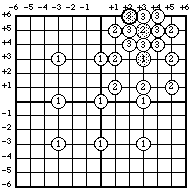## Three Step Search

At about the same time as Jain & Jain's introduction of the 2-D Logarithmic (TDL) search Koga et al. introduced a similar algorithm known as the Three Step Search (TSS) [Koga81]. As with the TDL search, a number of positions around a centre are tested and the position of minimum distortion becomes the centre of the next stage. The Three Step Search, however, tests eight points around the centre instead of four. For a centre [cx,cy] and step size s the positions [cx-s,cy-s ], [cx-s,cy ], [cx-s,cy+s ], [cx,cy-s ], [cx,cy ], [cx,cy+s ], [cx+s,cy-s ], [cx+s,cy ], [cx+s,cy+s ] are examined. After each stage the step size is reduced. Koga et al. illustrate the algorithm with a maximum displacement of ±6 pixels in both directions (as in Figure 3.9) with an initial step size of 3 and after each stage the step size is decremented. This allows the algorithm to halt in three steps and hence its name.The Three Step Search (TSS) converging on a position of minimum distortion at [+2,+6].

As with the TDL, there are variants of the TSS, and like the TDL they differ only in the manner in which the step size is decreased. The first variant (as per Koga et al.) reduces the step size by one after each stage. The second halves the step size after each stage.